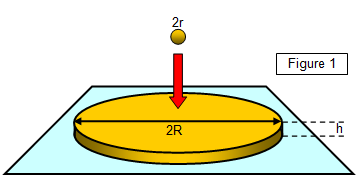# The oil drop experimentWe have considered the forces that exist in a solid between two adjacent molecules and we have therefore assumed the existence of these molecules.

The follow- ing simple experiment can be used to give a rough idea of the size of a molecule, and hence an atom.

The radius r of a small drop of oil is found and the volume of the drop calculated. The drop is now placed on the surface of some dust-covered water and the drop spreads out into a roughly circular patch of height h and radius R. The diameter of the patch is measured and hence the radius R is found.

Now the volume of the original drop (4/3πr3) is the same as that of the film (πr2h); therefore, if h is the thickness of the film and the original volume of the drop is known the thickness of the film can be found.

Now the thickness of the oil film cannot be less than the size of a molecule and so the size of a molecule of oil must be equal to or less than the thickness of the film.
If the thickness of the film (h) can be found this is the upper limit of the size of an oil molecule.

Example problem
A small drop of oil with a diameter of 0.15 mm is dropped onto a dust covered water surface. The resulting circular patch of oil has a diameter of 20 cm.
Calculate the maximum size of an oil molecule.

Volume of drop = 4/3 πr3 = 4/3 x π x (7.5x10-5)3 = 1.77x10-12 m3
Volume of circular patch = = πR2h = π x (0.1)2 x h

Therefore thickness oil film (h) = maximum size of an oil molecule = 0.6x10-10 m

Results from this experiment suggest that the diameter of a molecule of oil is about 10-10m, and this has been confirmed by X-ray diffraction.

The sizes of important particles may also be found from the following experiments:
(a) Size of nucleus – alpha particle scattering
(b) Size of atom – X ray diffraction
(c) Size of molecule – oil film